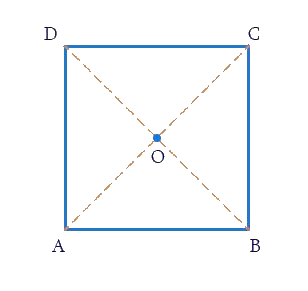# Ex.3.3 Q6 Understanding Quadrilaterals Solution-Ncert Maths Class 8

## Question

Two adjacent angles of a parallelogram have equal measure. Find the measure of each of the angles of the parallelogram.

Video Solution
Understanding Quadrilaterals
Ex 3.3 | Question 6

## Text Solution

What is Known?

Two adjacent angles of a parallelogram have equal measure.

What is Unknown?

Measure of each of the angles of the parallelogram.

Reasoning:

In parallelogram opposite angles are equal and adjacent angles are supplementary.

Using this property, we can calculate the unknown angles.

Steps:In parallelogram $$ABCD$$,

$$\angle {\rm{A }}$$ and $$\angle {\rm{D}}$$ are supplementary since $$DC$$ is parallel to $$AB$$ and with transversal $$DA$$,

making $$\angle {\rm{A}}$$ and $$\angle D$$ interior opposite.

$$\angle {\rm{A }}$$ and $$\angle {\rm{B }}$$ are also supplementary since $$AD$$ is parallel to $$BC$$ and with transversal $$BA$$, making $$\angle {\rm{A}}$$ and $$\angle {\rm{B}}$$ interior opposite.

Sum of adjacent angles $$= {\rm{18}}0^\circ$$

Let each adjacent angle be $$x$$

Since the adjacent angles in a parallelogram are supplementary.

\begin{align}x + x &= 180^\circ \\2x &= 180^\circ \\x &= \frac{{{\rm{18}}0^\circ }}{2}\end{align}

Hence, each adjacent angle is $$90$$.

\begin{align}\angle \text{A }&\!\!=\!\!\angle \text{B}\!\!=\!\!\text{ 9}0{}^\text{o}\text{ }\left( \text{adjacent angles} \right) \\ \angle \text{C }&\!\!=\!\!\angle \text{A}\!\!=\!\!\text{ 9}0{}^\text{o}\text{ }\left( \text{Opposite angles} \right) \\ \angle \text{D}&\!\!=\!\!\angle \text{B}\!\!=\!\!\text{9}0{}^\text{o}\left( \text{Opposite angles} \right) \end{align}

Thus, each angle of the parallelogram measures $$90^\circ$$.

Learn from the best math teachers and top your exams

• Live one on one classroom and doubt clearing
• Practice worksheets in and after class for conceptual clarity
• Personalized curriculum to keep up with school